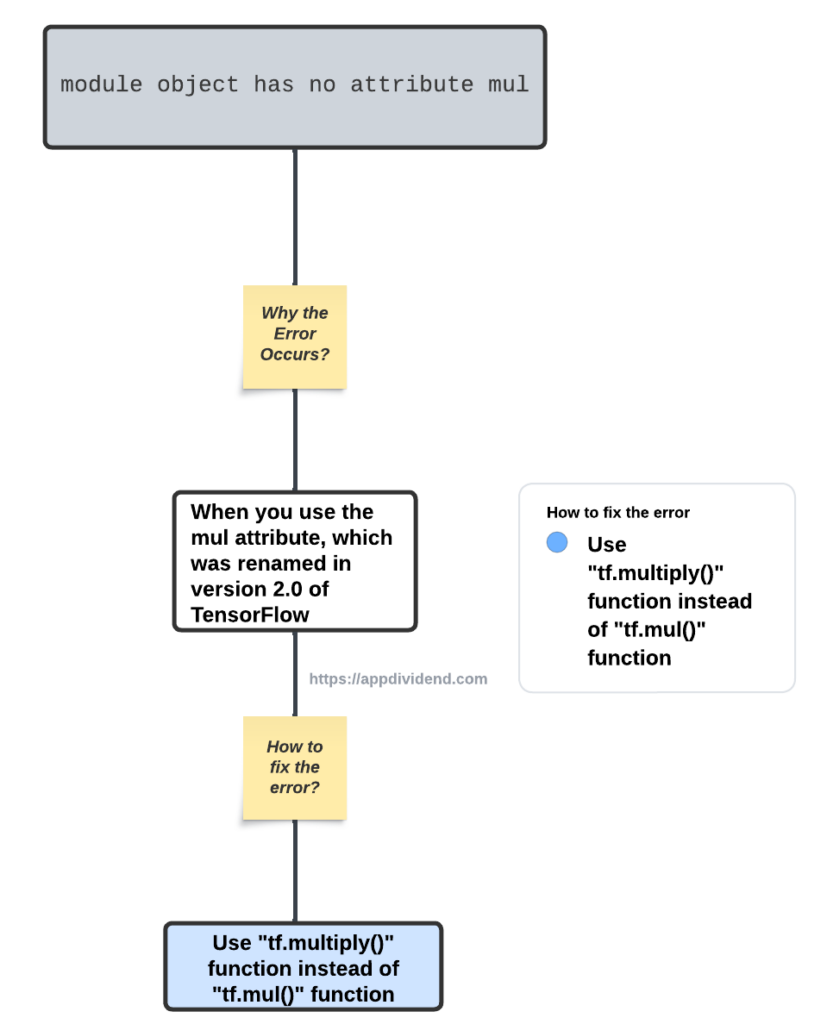# How to Fix AttributeError: ‘module’ object has no attribute ‘mul’Diagram

AttributeError: ‘module’ object has no attribute ‘mul’ error occurs because you “use the mul attribute, which was renamed in version 2.0 of TensorFlow.”

To fix the AttributeError: ‘module’ object has no attribute ‘mul’ error, use the “tf.multiply()” function instead of “tf.mul()” function.

## Reproduce the error

``````import tensorflow as tf

# Define two tensors
a = tf.constant([1, 2, 3])
b = tf.constant([4, 5, 6])

# Multiply the tensors element-wise
result = tf.mul(a, b)

# Print the result
print(result.numpy())
``````

Output## How to fix it?

Use tf.multiply instead of tf.mul, as the latter is not a valid attribute in the TensorFlow module (at least in recent versions). If you update your code to the tf.multiply() function, the error should be resolved!

``````import tensorflow as tf

# Define two tensors
a = tf.constant([1, 2, 3])
b = tf.constant([4, 5, 6])

# Multiply the tensors element-wise
result = tf.multiply(a, b)

# Print the result
print(result.numpy())
``````

Output

``[ 4 10 18]``

That’s it!

## Related posts

tf-trt warning: could not find tensorrt

AttributeError: module ‘tensorflow’ has no attribute ‘test’

AttributeError: module ‘tensorflow’ has no attribute ‘variable_scope’

AttributeError: module ‘tensorflow’ has no attribute ‘layers’

AttributeError: ‘Sequential’ Object Has No Attribute ‘predict_classes’

AttributeError: module ‘tensorflow’ has no attribute ‘ConfigProto’

This site uses Akismet to reduce spam. Learn how your comment data is processed.